# Isotropic Biased and Orthotropic Analog PlatesThe purpose of this test is to validate orthotropic elements properties. You will use an isotropic biased plate and an orthotropic analog plate, computed with a 2D mesh.Reference:

M. JONES, Mechanics of Composite Materials, Hemisphere publishing corporation, pp250-254, 1975.

ASHTON JE, An Analogy for Certain Anisotropic Plate, Journal of Composite Materials, pp355-358, April 1969.

##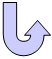Specifications

### Geometry Specifications

 Isotropic biased plate: Length: L = 500 mm Thickness: th = 10 mm Angle: θ= 60 deg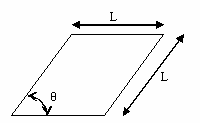Isotropic plate Orthotropic analog plate: Length: L = 500 mm Thickness: th = 10 mm Square geometry with principal axes inclined at -45 deg   with respect to the lower side.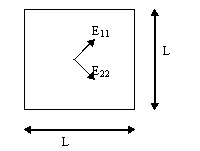Orthotropic plate

### Analysis Specifications

 Isotropic plate: Young Modulus (material): E = 1000 MPa Poisson's Ratio: ν = 0 Loads (pressure): P1 = 1000 Pa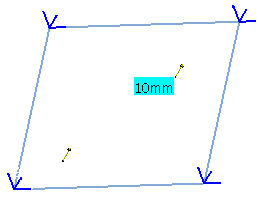Orthotropic plate: Young Modulus (material): E11 = 2250 MPa E22 = 250 MPa Shear Modulus: G12 = 375 MPa Poisson's Ratio: ν12 = 0 Loads (pressure): P2 = 562.5 Pa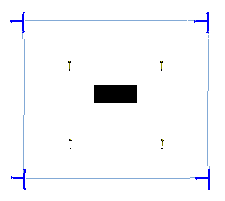Mesh Specifications: 13 x 13 nodes Restraints (User defined): Tx = Ty = Tz = 0 for all edges

##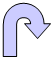Results

• Analytical Solution:

The following formula gives the analytical solution for the vertical displacement at the center of an isotropic biased plate: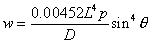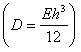This solution is also valid for an orthotropic plate with the geometry specifications indicated below, and with characteristics given by: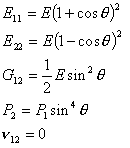• Computed Results:

The computed results (in mm) are given in the tables below.
Normalized results (computed results divided by analytical solution) are listed. Plates Analytical Solution Linear Triangle (TR3) Parabolic Triangle (TR6) Linear Quadrangle (QD4) Parabolic Quadrangle (QD8)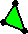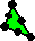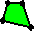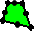Computed Results Normalized Results Computed Results Normalized Results Computed Results Normalized Results Computed Results Normalized Results Biased Isotropic 1.907 1.988 1.048 2.011 1.055 1.933 1.013 1.977 1.037 Analog Orthotropic 1.907 1.900 0.996 1.931 1.012 1.908 1.001 1.884 0.988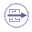To Perform the Test:

The Isotropic_biased_plate_QD4.CATAnalysis document presents the complete analysis on an isotropic biased plate, computed with a mesh formed of linear quadrangle elements (QD4).

The Orthotropic_analog_plate_QD4.CATAnalysis document presents a complete analysis on an orthotropic analog plate, computed with a mesh formed of linear quadrangle elements (QD4).

To compute the case with other meshes (TR3, TR6 and QD8), proceed as follow:

1. Open one of the CATAnalysis documents.

2. In the Advanced Meshing Tools workbench, replace the mesh specifications as indicated above.

3. In the Generative Structural Analysis workbench, compute the case.